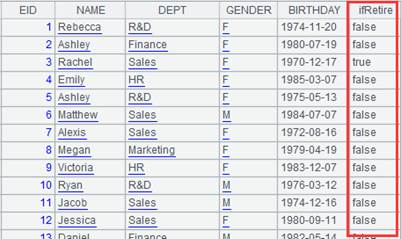# isect()

## A.isect()

A.isect()

 A 成员为序列的序列

 A 1 =[[1,2,3,4,5],[3,7,8]].isect()  2 =[[1,2,3],[3,2]].isect() [2,3] 3 =[[1,2,2,3],2].isect()  4 =demo.query("select top 2 * from EMPLOYEE")5 =demo.query("select top 1 * from EMPLOYEE")6 =[A4,A5].isect() []，因为A4和A5来自不同序表，存储地址不同，因此相同记录视为不同的成员

A.conj()

A.xunion()

## A.isect(x)

A.isect(x)

 A 成员为序列的序列 x 表达式，返回序列

 A 1 =demo.query("select * from EMPLOYEE where GENDER = 'M' order by NAME") 2 =demo.query("select * from EMPLOYEE where GENDER = 'F' order by NAME") 3 =[A1,A2].isect(~.(NAME)) A1,A2交列运算

A.isect()